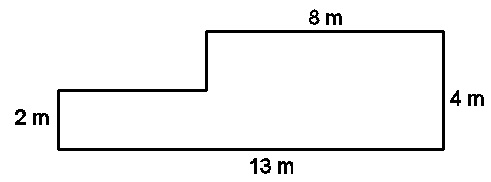# Calculate mentally

Geometry Level 1Calculate the area of the figure above mentally. Give your answer in square meters.

Note : All corner angles are 90 degrees.

×